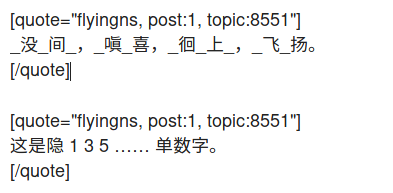# 如何实现「把一段文字每隔一个字就隐藏一个字」？（内容有改动）

Perl 5 还是 6 啊

``````(defun my-hide (beg end)
(interactive "r")
(let ((pt beg)
(i 1))
(while (<= pt end)
(when (aref (char-category-set (char-after pt)) ?C)
(when (cl-evenp i) ; `cl-evenp'用来隐双数字，`cl-oddp'用来隐单数字。
(put-text-property pt (1+ pt) 'display
;; 原地替换＂离＂为＂_＂、＂*＂或者＂[离]＂。
(concat "[" (char-to-string (char-after pt)) "]")))
(cl-incf i))
(cl-incf pt))))
``````

org-drill要求是 [啊]

text-property可没用

https://paste.ubuntu.com/p/mGQB7Mf2t4/

2 个赞

``````(cm/mask-string "易学看人啊，不置可否。" nil t)

"[易]学[看]人[啊]，不[置]可[否]。"
``````

1 个赞

Today I learned 写正则是程序员约架的常用方式

1 个赞``````(defun cm/mask-string (s &optional mask-even-p by-word-p count-in-total)
(let ((one-word (rx (or (and bow (1+ (any "a-z" "A-Z" "0-9")))
(category chinese-two-byte))))
(punct (rx (or punct blank))))
(-let (((contents seps) (cm/split* punct s 'omit-null))
(pred (if mask-even-p #'cl-evenp #'cl-oddp)))
(setq contents (if by-word-p
(-let [total-idx 0]
(--map
(->> it
(s-match-strings-all one-word)
(-flatten)
(-map-indexed
(lambda (idx subs)
(if (funcall pred (if count-in-total
(setq total-idx (1+ total-idx))
(1+ idx)))
(s-wrap subs "[" "]")
subs)))
(s-join ""))
contents))
(--map-indexed
(if (funcall pred (1+ it-index))
(s-wrap it "[" "]")
it)
contents)))
(s-join "" (apply #'-interleave (-pad "" contents seps))))))
``````

1 个赞